# Lecture 4 - Growth of Functions (1).ppt

27 May 2023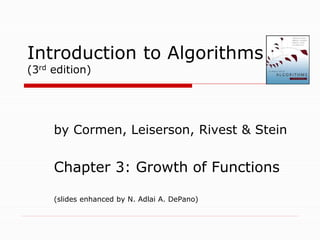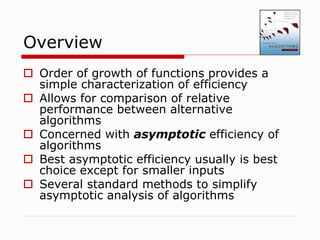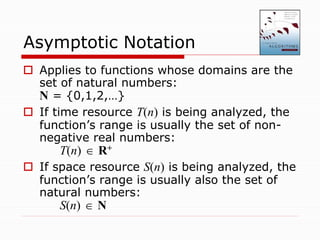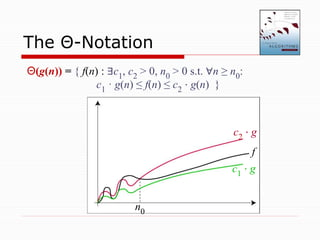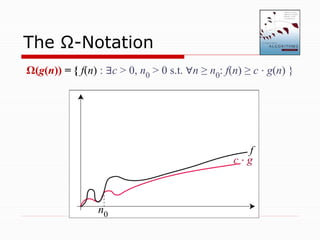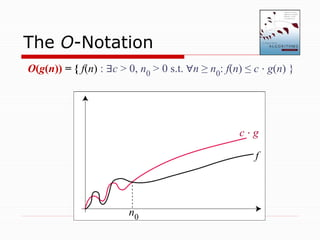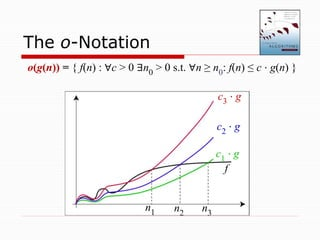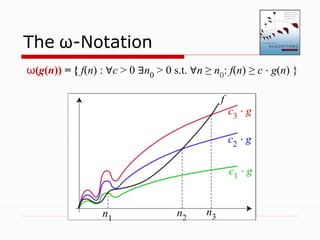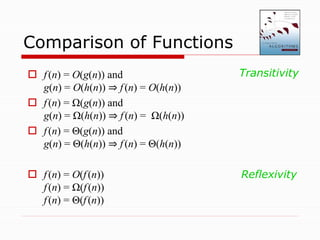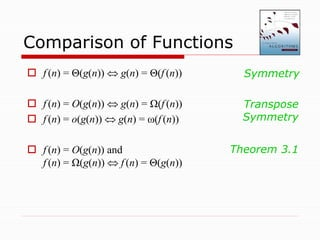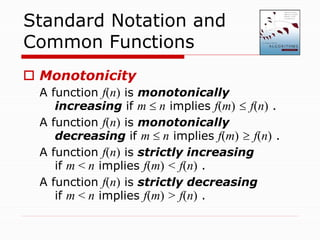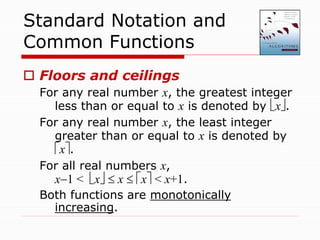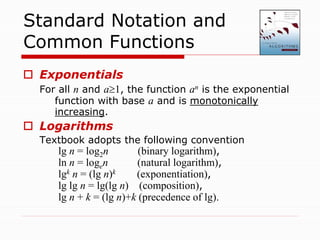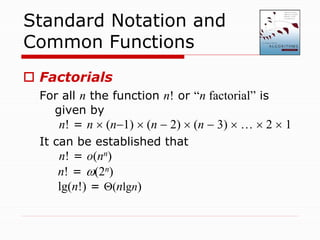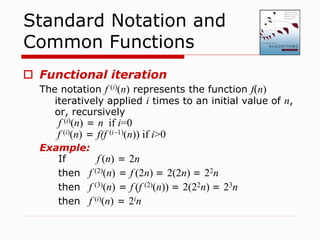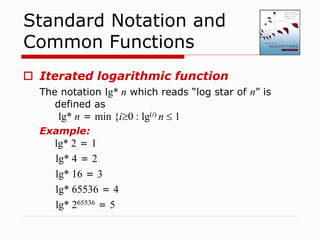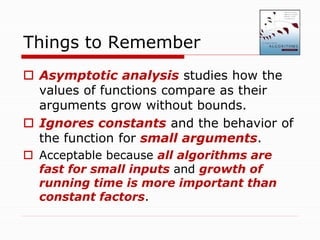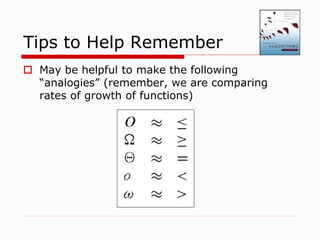1 sur 20

### Lecture 4 - Growth of Functions (1).ppt

• 1. Introduction to Algorithms (3rd edition) by Cormen, Leiserson, Rivest & Stein Chapter 3: Growth of Functions (slides enhanced by N. Adlai A. DePano)
• 2. Overview  Order of growth of functions provides a simple characterization of efficiency  Allows for comparison of relative performance between alternative algorithms  Concerned with asymptotic efficiency of algorithms  Best asymptotic efficiency usually is best choice except for smaller inputs  Several standard methods to simplify asymptotic analysis of algorithms
• 3. Asymptotic Notation  Applies to functions whose domains are the set of natural numbers: N = {0,1,2,…}  If time resource T(n) is being analyzed, the function’s range is usually the set of non- negative real numbers: T(n)  R+  If space resource S(n) is being analyzed, the function’s range is usually also the set of natural numbers: S(n)  N
• 4. Asymptotic Notation  Depending on the textbook, asymptotic categories may be expressed in terms of -- a. set membership (our textbook): functions belong to a family of functions that exhibit some property; or b. function property (other textbooks): functions exhibit the property  Caveat: we will formally use (a) and informally use (b)
• 5. The Θ-Notation f c1 ⋅ g n0 c2 ⋅ g Θ(g(n)) = { f(n) : ∃c1, c2 > 0, n0 > 0 s.t. ∀n ≥ n0: c1 · g(n) ≤ f(n) ≤ c2 ⋅ g(n) }
• 6. The Ω-Notation Ω(g(n)) = { f(n) : ∃c > 0, n0 > 0 s.t. ∀n ≥ n0: f(n) ≥ c ⋅ g(n) } f c ⋅ g n0
• 7. The O-Notation f c ⋅ g n0 O(g(n)) = { f(n) : ∃c > 0, n0 > 0 s.t. ∀n ≥ n0: f(n) ≤ c ⋅ g(n) }
• 8. The o-Notation o(g(n)) = { f(n) : ∀c > 0 ∃n0 > 0 s.t. ∀n ≥ n0: f(n) ≤ c ⋅ g(n) } f c1 ⋅ g n1 c2 ⋅ g c3 ⋅ g n2 n3
• 9. The ω-Notation f c1 ⋅ g n1 c2 ⋅ g c3 ⋅ g n2 n3 ω(g(n)) = { f(n) : ∀c > 0 ∃n0 > 0 s.t. ∀n ≥ n0: f(n) ≥ c ⋅ g(n) }
• 10.  f(n) = O(g(n)) and g(n) = O(h(n)) ⇒ f(n) = O(h(n))  f(n) = Ω(g(n)) and g(n) = Ω(h(n)) ⇒ f(n) = Ω(h(n))  f(n) = Θ(g(n)) and g(n) = Θ(h(n)) ⇒ f(n) = Θ(h(n))  f(n) = O(f(n)) f(n) = Ω(f(n)) f(n) = Θ(f(n)) Comparison of Functions Reflexivity Transitivity
• 11.  f (n) = Θ(g(n))  g(n) = Θ(f (n))  f (n) = O(g(n))  g(n) = Ω(f (n))  f (n) = o(g(n))  g(n) = ω(f (n))  f (n) = O(g(n)) and f (n) = Ω(g(n))  f (n) = Θ(g(n)) Comparison of Functions Transpose Symmetry Symmetry Theorem 3.1
• 12. Standard Notation and Common Functions  Monotonicity A function f(n) is monotonically increasing if m  n implies f(m)  f(n) . A function f(n) is monotonically decreasing if m  n implies f(m)  f(n) . A function f(n) is strictly increasing if m < n implies f(m) < f(n) . A function f(n) is strictly decreasing if m < n implies f(m) > f(n) .
• 13. Standard Notation and Common Functions  Floors and ceilings For any real number x, the greatest integer less than or equal to x is denoted by x. For any real number x, the least integer greater than or equal to x is denoted by x. For all real numbers x, x1 < x  x  x < x+1. Both functions are monotonically increasing.
• 14. Standard Notation and Common Functions  Exponentials For all n and a1, the function an is the exponential function with base a and is monotonically increasing.  Logarithms Textbook adopts the following convention lg n = log2n (binary logarithm), ln n = logen (natural logarithm), lgk n = (lg n)k (exponentiation), lg lg n = lg(lg n) (composition), lg n + k = (lg n)+k (precedence of lg).
• 15. Standard Notation and Common Functions  Factorials For all n the function n! or “n factorial” is given by n! = n  (n1)  (n  2)  (n  3)  …  2  1 It can be established that n! = o(nn) n! = (2n) lg(n!) = (nlgn)
• 16.  Functional iteration The notation f (i)(n) represents the function f(n) iteratively applied i times to an initial value of n, or, recursively f (i)(n) = n if i=0 f (i)(n) = f(f (i1)(n)) if i>0 Example: If f (n) = 2n then f (2)(n) = f (2n) = 2(2n) = 22n then f (3)(n) = f (f (2)(n)) = 2(22n) = 23n then f (i)(n) = 2in Standard Notation and Common Functions
• 17.  Iterated logarithmic function The notation lg* n which reads “log star of n” is defined as lg* n = min {i0 : lg(i) n  1 Example: lg* 2 = 1 lg* 4 = 2 lg* 16 = 3 lg* 65536 = 4 lg* 265536 = 5 Standard Notation and Common Functions
• 18.  Asymptotic analysis studies how the values of functions compare as their arguments grow without bounds.  Ignores constants and the behavior of the function for small arguments.  Acceptable because all algorithms are fast for small inputs and growth of running time is more important than constant factors. Things to Remember
• 19.  Ignoring the usually unimportant details, we obtain a representation that succinctly describes the growth of a function as its argument grows and thus allows us to make comparisons between algorithms in terms of their efficiency. Things to Remember
• 20. Tips to Help Remember  May be helpful to make the following “analogies” (remember, we are comparing rates of growth of functions)